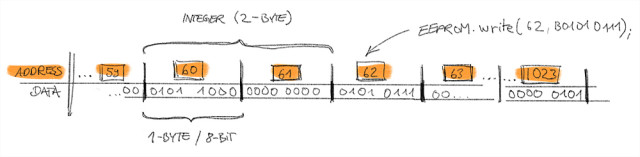﻿ Write an integer to the Arduino EEPROM – Electronics Scriblab

## Write an integer to the Arduino EEPROM

Today I faced the problem that I wanted to write an int (integer) to the integrated Arduino EEPROM. The Atmel ATMega328 chip has an address space of 0-1023 and each of these slots can save 1-Byte or 8-Bit (which is the same btw).

Now an int is a number that goes from −32.768 to 32.767 (or 65.535 if unsigned) and needs 2 Bytes for storage because this range of values can be decoded in 2-Bytes or 16-Bits (eg. 1100 0000 0101 0001 = 49.233)

The thing is that the standart Arduino EEPROM library just writes 1 Byte (values from 0-255 or a number that can be decoded in 8-Bit (1-Byte) eg. “0000 0110”) to an address specified.

Here is a little illustration how the EEPROM (or our little problem) “looks” like:So I did some research and found some solutions. All you need to do is to do some bit-shifting magic and voilá you can decompose the int to its Byte components (taking the first and the last 8-Bits) and save it to actually 2 addresses.

Here is a code snippet of two functions you can use for writing and reading integers to the Arduino EEPROM:

Instead of the EEPROM.write() method of the EEPROM library I used the EEPROM.update() method.

The advantage is that the value that is stored at the EEPROM address is only written when the value is actually different from the current value that is stored at that adress. If the value is the same, the value is not writen at all which save us some write-cycles in the EEPROM slot (it’s limited to 100.000 write cycle per adress) and execution time (3.3ms when the Arduino actually writes something).

You need to be aware of the fact, that the int occupies 2 Bytes in the EEPROM. So if you write an int to the adress “60” the next free slot you can use would be 60+2 = 62. The int occupies byte 60 and byte 61 in the EEPROM.

Happy EEPROM writing!

Merken

Merken

Merken

11. December 2016 by Marius